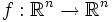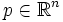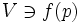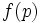Inverse function theorem

Let$f:\R^n \to \R^n$ be a differentiable map such that the determinant of the Jacobian matrix of$f$ at a particular point$p \in \R^n$, is nonzero (i.e. the Jacobian matrix is nonsingular). Then, there exist open neighbourhoods$U \ni p$ and$V \ni f(p)$ such that the restriction of$f$ to$U$ is a diffeomorphism from$p$ to$f(p)$.